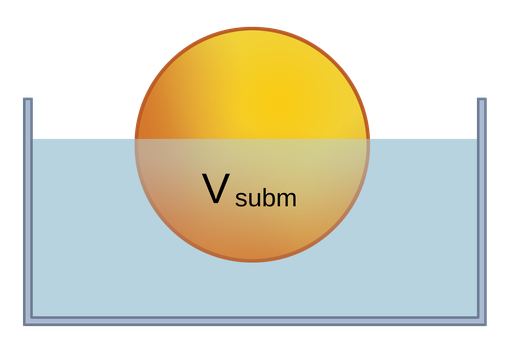We have seen that if an object’s average density is less than that of the liquid it is placed in, it will rise to the surface and float. We can use the concept of equilibrium to show that for a floating object, the buoyant force balances its weight, , i.e., FB = m · g.

Since the object is less dense than the fluid, only part of its volume - the submerged volume - is under the surface, so we can write the buoyant force from Archimedes principle as,

FB = ρfluid · Vsubm · g.

The object’s weight mobject· g can be written as:

mobject· g = ρobject · Vobject · g.

Setting this equation for a floating object,

ρfluid · Vsubm · g = ρobject · Vobject · g,

then canceling the “g” and rearranging, we find that the ratio of submerged to total volume, the submerged fraction, is

Vsubm/Vobject = ρobjectfluid.

That is, the submerged fraction of a floating object is equal to the ratio of its density to that of the fluid. This makes sense - a less dense object will have a lower submerged fraction and will therefore float “higher”in the liquid.Figure 1:A floating object, showing the submerged volume below the surface. For this example, we can tell that since the submerged fraction is between 0.5 and 1.0, the object’s density relative to that of the liquid must be between 0.5 and 1.0.